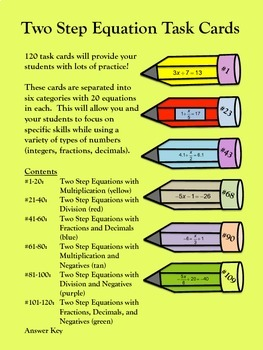Two Step Equation Task Cards (Digital/PDF)7th - 10th, Homeschool
Subjects
Standards
Resource Type
Formats Included
• Zip
•Google Apps™
Pages
17 pagesThe Teacher-Author indicated this resource includes assets from Google Workspace (e.g. docs, slides, etc.).

Also included in

1. This download includes both a PDF and digital Google Forms version of this activity.This bundle contains 360 task cards!Both color and black & white cards are included.Some of the "One Step Equation Task Cards" can be used in 4th or 5th grade to practice computation skills. Solving one step equa
\$10.00
\$15.00
Save \$5.00

Description

Both color and black & white cards are included.

These cards are separated into six categories with 20 equations in each. This will allow you and your students to focus on specific skills while using a variety of types of numbers (integers, fractions, decimals).

Contents

#1-20: Two Step Equations with Multiplication (yellow)

#21-40: Two Step Equations with Division (red)

#41-60: Two Step Equations with Fractions and Decimals (blue)

#61-80: Two Step Equations with Multiplication and Negatives (tan)

#81-100: Two Step Equations with Division and Negatives (purple)

#101-120: Two Step Equations with Fractions, Decimals, and Negatives (green)

Check out One Step Equation Task Cards and Multi Step Equation Task Cards as well!

Here are some other two step equation activities:

Total Pages
17 pages
Included
Teaching Duration
N/A
Report this Resource to TpT
Reported resources will be reviewed by our team. Report this resource to let us know if this resource violates TpT’s content guidelines.

Standards

to see state-specific standards (only available in the US).
Solve linear equations in one variable.
Solve word problems leading to equations of the form 𝘱𝘹 + 𝘲 = 𝘳 and 𝘱(𝘹 + 𝘲) = 𝘳, where 𝘱, 𝘲, and 𝘳 are specific rational numbers. Solve equations of these forms fluently. Compare an algebraic solution to an arithmetic solution, identifying the sequence of the operations used in each approach. For example, the perimeter of a rectangle is 54 cm. Its length is 6 cm. What is its width?
Use variables to represent quantities in a real-world or mathematical problem, and construct simple equations and inequalities to solve problems by reasoning about the quantities.